# Using a net to find the surface area of a rectangular prism Online Quiz

Following quiz provides Multiple Choice Questions (MCQs) related to Using a net to find the surface area of a rectangular prism. You will have to read all the given answers and click over the correct answer. If you are not sure about the answer then you can check the answer using Show Answer button. You can use Next Quiz button to check new set of questions in the quiz.Q 1 - Find the surface area of the given rectangular prism in square cm.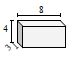### Explanation

Step 1:

Using net, the surface area of a rectangular prism = 2(lw + wh + lh); l = 8 ; w = 4; h = 3

Step 2:

Surface area of given prism = 2(8×4 + 8×3 + 3×4)

= 2(32 + 24 + 12)

= 136 square cm

Q 2 - Find the surface area of the given rectangular prism in square cm.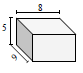### Explanation

Step 1:

Using net, the surface area of a rectangular prism = 2(lw + wh + lh); l = 8 ; w = 5; h = 9

Step 2:

Surface area of given prism = 2(8×5 + 8×9 + 5×9)

= 2(40 + 72 + 45)

= 314 square cm

Q 3 - Find the surface area of the given rectangular prism in square cm.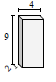### Explanation

Step 1:

Using net, the surface area of a rectangular prism = 2(lw + wh + lh); l = 4 ; w = 9; h = 2

Step 2:

Surface area of given prism = 2(9×4 + 4×2 + 9×2)

= 2(36 + 8 + 18)

= 124 square cm

Q 4 - Find the surface area of the given rectangular prism in square cm.### Explanation

Step 1:

Using net, the surface area of a rectangular prism = 2(lw + wh + lh); l = 2 ; w = 3; h = 8

Step 2:

Surface area of given prism = 2(2×3 + 8×2 + 3×8)

= 2(6 + 16 + 24)

= 92 square cm

Q 5 - Find the surface area of the given rectangular prism in square cm.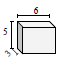### Explanation

Step 1:

Using net, the surface area of a rectangular prism = 2(lw + wh + lh); l = 6 ; w = 5; h = 3

Step 2:

Surface area of given prism = 2(6×5 + 6×3 + 3×5)

= 2(30 + 18 + 15)

= 126 square cm

Q 6 - Find the surface area of the given rectangular prism in square cm.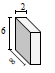### Explanation

Step 1:

Using net, the surface area of a rectangular prism = 2(lw + wh + lh); l = 3 ; w = 6; h = 8

Step 2:

Surface area of given prism = 2(3×6 + 8×3 + 6×8)

= 2(18 + 24 + 48)

= 180 square cm

Q 7 - Find the surface area of the given rectangular prism in square cm.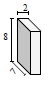### Explanation

Step 1:

Using net, the surface area of a rectangular prism = 2(lw + wh + lh); l = 2 ; w = 8; h = 7

Step 2:

Surface area of given prism = 2(8×2 + 2×7 + 8×7)

= 2(16 + 14 + 56)

= 172 square cm

Q 8 - Find the surface area of the given rectangular prism in square cm.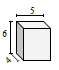### Explanation

Step 1:

Using net, the surface area of a rectangular prism = 2(lw + wh + lh); l = 5 ; w = 6; h = 4

Step 2:

Surface area of given prism = 2(5×6 + 5×4 + 6×4)

= 2(30 + 20 + 24)

= 148 square cm

Q 9 - Find the surface area of the given rectangular prism in square cm.### Explanation

Step 1:

Using net, the surface area of a rectangular prism = 2(lw + wh + lh); l = 6 ; w = 9; h = 6

Step 2:

Surface area of given prism = 2(6×9 + 6×6 + 6×9)

= 2(54 + 36 + 54)

= 288 square cm

Q 10 - Find the surface area of the given rectangular prism in square cm.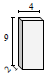### Explanation

Step 1:

Using net, the surface area of a rectangular prism = 2(lw + wh + lh); l = 4 ; w = 9; h = 2

Step 2:

Surface area of given prism = 2(4×9 + 4×2 + 9×2)

= 2(36 + 8 + 18)

= 124 square cm

using_net_to_find_surface_area_of_rectangular_prism.htm